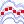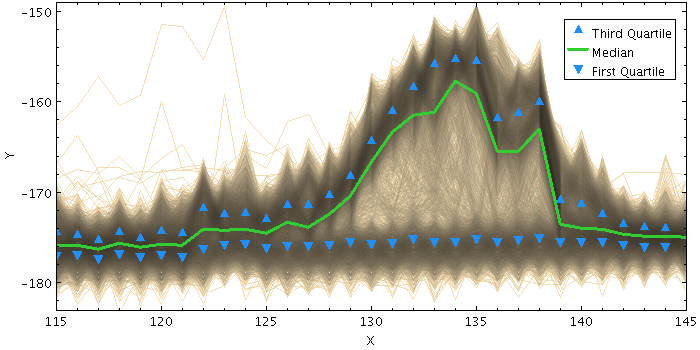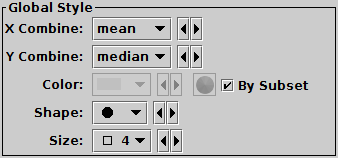Next Previous Up Contents
Next: ArrayQuantile Form
Up: Plot Forms
Previous: StatLine Form

#### A.4.5.38 StatMark Form

The StatMark form (), available from the XYArray Layer Control, plots a set of markers based on a combination (typically the mean) of input array-valued coordinates. The input X and Y coordinates must be fixed-length arrays of length N; N markers are plotted, each one representing the mean (or median, minimum, maximum, ...) of all the input array elements at the corresponding position.

Note that because the X and Y arrays must be of a fixed size for all rows, and because combination is performed in both X and Y directions, this is typically only suitable for plotting combined spectra if they all share a common horizontal axis, e.g. are all sampled into the same wavelength bins. To visually combine spectra with non-uniform sampling, the ArrayQuantile form may be more useful.Example StatMark plotStatLine form configuration panel

The configuration options are:

X Combine
Y Combine
Defines how corresponding array elements on the X/Y axis are combined together to produce the plotted value. The following options are currently available:
• `mean`: the mean of the combined values
• `median`: the median of the combined values (may be slow)
• `min`: the minimum of all the combined values
• `max`: the maximum of all the combined values
• `q.01`: the 1st percentile of the combined values (may be slow)
• `q1`: the first quartile of the combined values (may be slow)
• `q3`: the third quartile of the combined values (may be slow)
• `q.99`: the 99th percentile of the combined values (may be slow)
• `stdev`: the sample standard deviation of the combined values
• `sum`: the sum of all the combined values
• `count`: the number of non-blank values
Color
Color of the plotted markers.
Shape
Shape of the plotted markers.
Size
Size of the plotted markers in pixels.

Next Previous Up Contents
Next: ArrayQuantile Form
Up: Plot Forms
Previous: StatLine Form

TOPCAT - Tool for OPerations on Catalogues And Tables